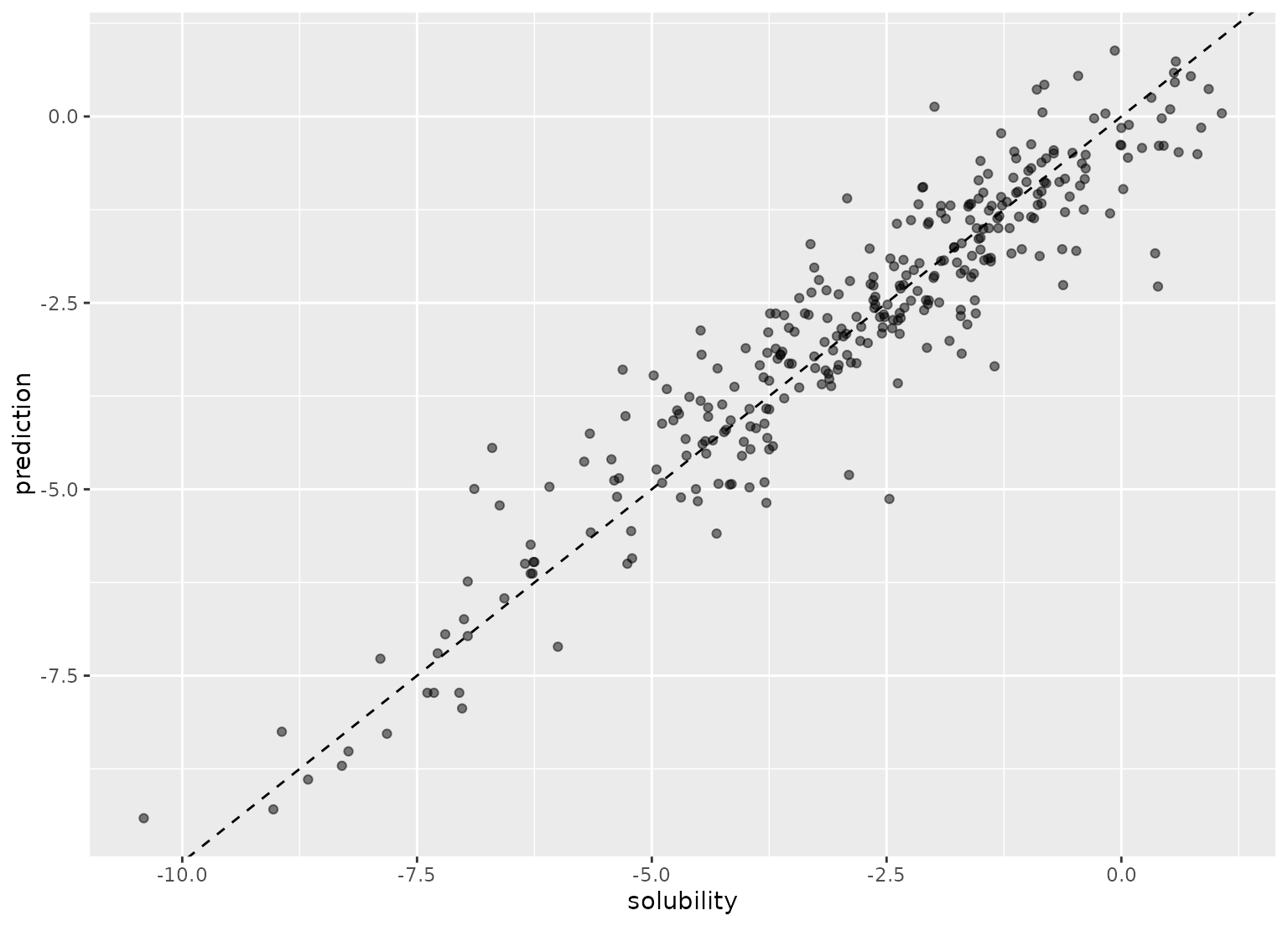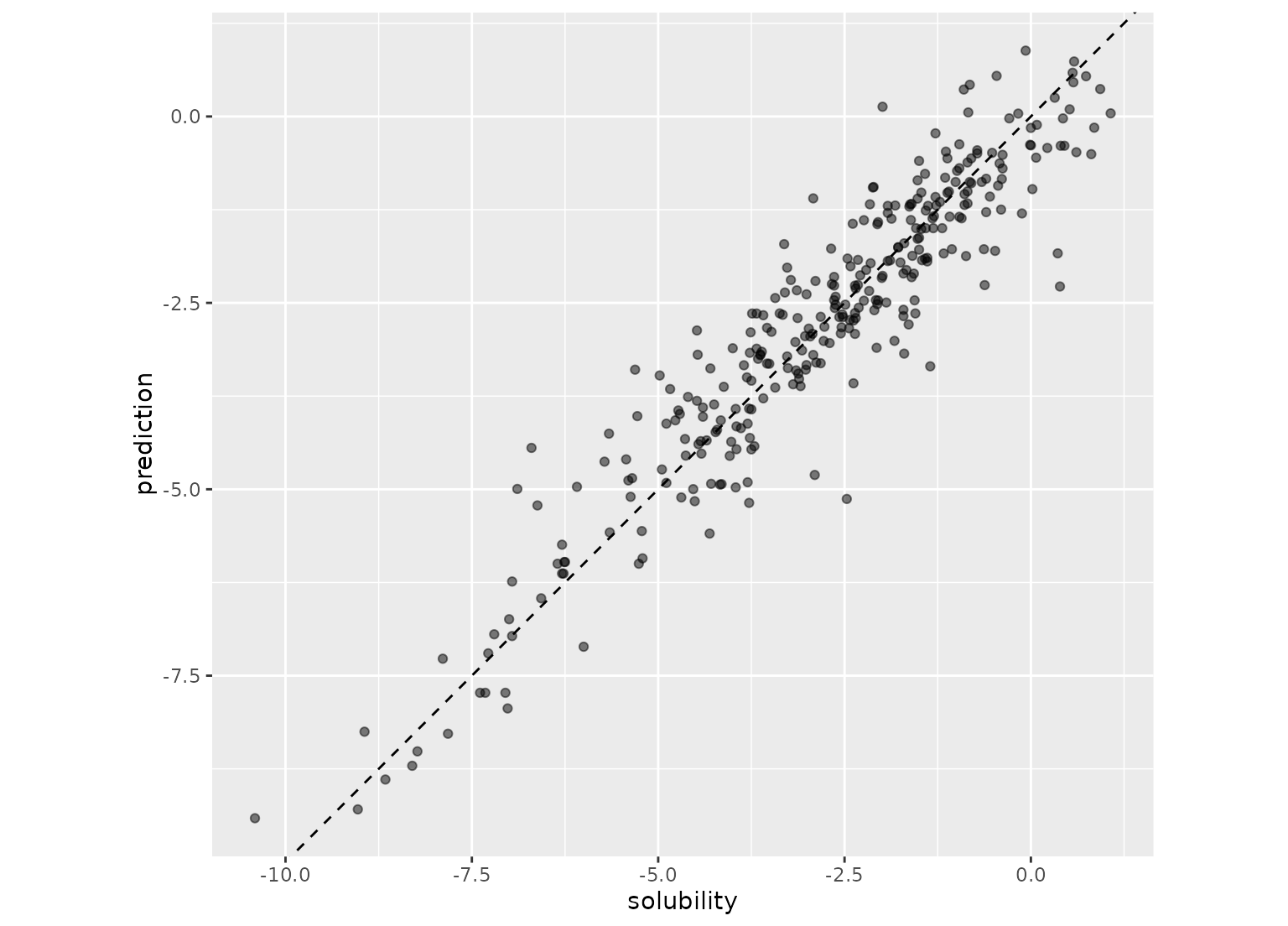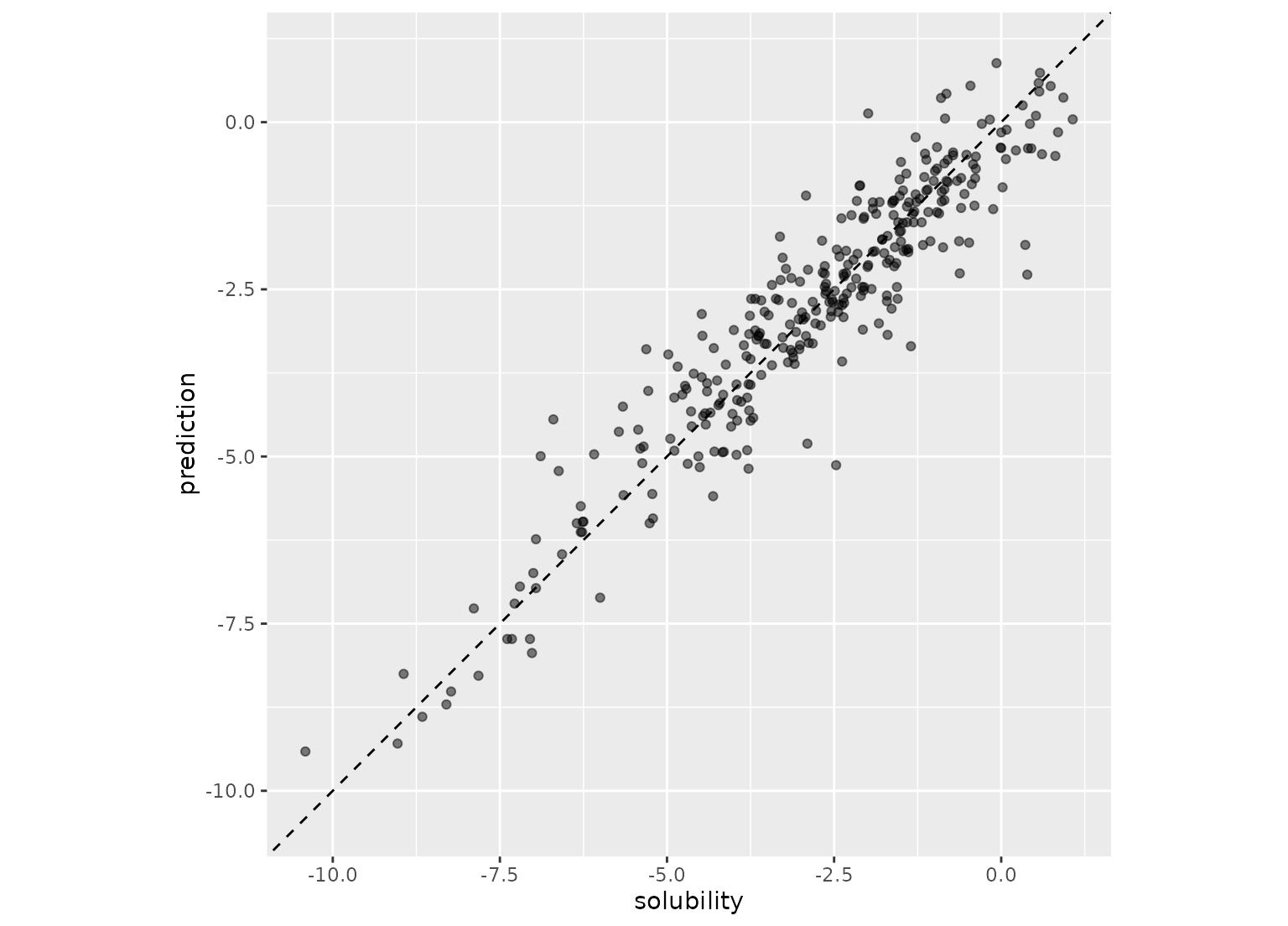For regression models, coord_obs_pred() can be used in a ggplot to make the x- and y-axes have the same exact scale along with an aspect ratio of one.

## Usage

coord_obs_pred(ratio = 1, xlim = NULL, ylim = NULL, expand = TRUE, clip = "on")

## Arguments

ratio

Aspect ratio, expressed as y / x. Defaults to 1.0.

xlim, ylim

Limits for the x and y axes.

expand

Not currently used.

clip

Should drawing be clipped to the extent of the plot panel? A setting of "on" (the default) means yes, and a setting of "off" means no. In most cases, the default of "on" should not be changed, as setting clip = "off" can cause unexpected results. It allows drawing of data points anywhere on the plot, including in the plot margins. If limits are set via xlim and ylim and some data points fall outside those limits, then those data points may show up in places such as the axes, the legend, the plot title, or the plot margins.

## Value

A ggproto object.

## Examples

data(solubility_test, package = "modeldata")

library(ggplot2)
p <- ggplot(solubility_test, aes(x = solubility, y = prediction)) +
geom_abline(lty = 2) +
geom_point(alpha = 0.5)

pp + coord_fixed()p + coord_obs_pred()